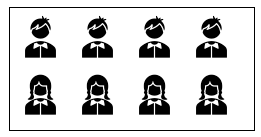Search
•Sai Geetha M N

# Decision Trees - How to decide the split?

In the introduction to Decision trees, we have seen that the whole process is to keep splitting one node into two based on certain features and feature values.

The idea of the split is to ensure that the subset is more pure or more homogeneous after the split.

There are two aspects we need to understand here:

1. The concept of homogeneity or purity - what does it mean?

2. How do we measure purity or impurity?

Only then, we can use this for splitting the nodes correctly.

## Homogeneity

Let us take an example to understand this concept.

Take a look at these two sets of data:Dataset ADataset B

In dataset A, we have 4 boys and 4 girls. An equal number of both genders. This means that this data set is a complete mix or completely non-homogenous as there is a big ambiguity about which gender this data set represents.

However, if you look at dataset B, you will see that all are girls. there is no ambiguity at all. This is, therefore, said to be a completely homogenous dataset with 100% purity or no impurity at all.

And all data could lie somewhere in between these two levels of impurity: Totally impure to totally pure.

This concept, though it seems trivial or pretty obvious, becomes the foundation stone on which decision trees are built and hence I thought of calling this out explicitly.

Since decision trees can be used for both classification and regression problems, the homogeneity has to be understood for both. For classification purposes, a data set is completely homogeneous if it contains only a single class label. For regression purposes, a data set is completely homogeneous if its variance is as small as possible

### How does this concept help?

1. We want to be able to split in such a way that the new groups formed are as homogenous as possible. We are not mandating a 100% purity but intend to move towards more purity than the parent node.

2. And we will see later (in another post) that the variable or feature we use for splitting also uses this concept of increasing homogeneity.

3. Going further, this concept also helps in determining the importance of a feature to an outcome, a very useful aspect when we want to take actions at a feature level.

## Purity or Impurity Measurements

There are various methodologies that are used for measuring the homogeneity of a node.

The two commonly used ones are:

• Gini Index &

• Entropy

But to better understand this concept, I will also look at another method by name "Classification Error" or Misclassification measure

This is mostly never used in real life problems but helps clarify the concept of an impurity measure with ease.

#### Classification Error

This is the error we see when we assign a majority class label to a node. The number or probability of the whole minority data points being misclassified is called a classification error.

Therefore, the formula for the classification error is:We will understand this with an example in the next post.

Similarly, I will share the formula for Gini Index and Entropy as well here but get into details with examples in the next post

#### Gini Index

This is defined as the sum of p(1-p) where p is the probability of each class and is represented better as:where there are K number of classes

#### Entropy

This concept has come from Physics - in particular thermodynamics where entropy is defined as a measure of randomness or disorder of a system. And in some sense that is what we are also trying to measure when we look for impurity.

Here, it is defined as the sum of p*logp (log to the base 2) where p is the probability of each class in that node. It is represented as:What do these formula convey is something that we can get into with some examples next week and understand how each one of these measures help in meaning the purity or the impurity of a node.